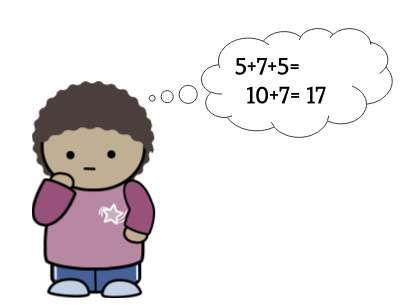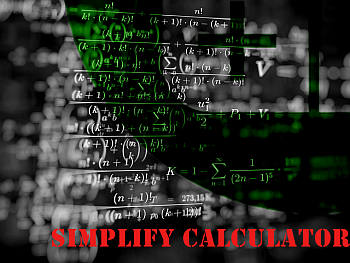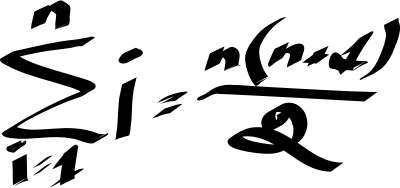# Simplify Calculator

Instructions: Use this simplify calculator to simplify any valid algebraic expression, either numeric or symbolic. Please type in the expression you want to simplify in the form box below.Enter the expression you want to simplify (Ex: 2x/3 + 4/5, etc.)

## Simplify Expressions Calculator

This simplify calculator with steps will allow you to simplify expressions that you provide, showing all the steps. You need to provide a valid expression that is either numeric or symbolic. For example, a valid numeric expression is something like 1/3+1/4*3^2, and a valid symbolic expression could be something like x^2 - 2x + 3/4 x +2', or maybe something like '(x^2-1)(x-1)', just to give an example.

Once you provide a valid expression, all you need to do is click the "Calculate" button that is right below, and you all the relevant steps of the process will be shown to you.

Some simplifications are easier to conduct than others. Some expression lend themselves easily to be simplified, others don't. Some algebraic expressions will require extensive and laborious steps to be simplified, and others just cannot be simplified.## How to Simplify?

Simplifying is not necessarily a simple process that consists of grouping terms together in an aim of shortening the given expression. The grouping process though is not arbitrary and it follows some strict rules and restrictions, that can be summarized in 6 letters: PEMDAS. We have:

P = Parentheses

E = Exponents

M = Multiplication

D = Division

S = Subtraction

So, an expression is composed by elements like numbers or unknown variables like 'x' that represent a number, and different operations that combine them. PEMDAS show us which operations should be conducted first. This is, you work on parentheses first, then on the exponents, then you do the multiplications and so on.

## What are the steps for simplifying expressions

• Step 1: Identify the expression you need to simplify. A valid expression needs to contain numbers and symbols like 'x' (that represent numbers)
• Step 2: Check for the consistency of the expression. This is, make sure that any opening parenthesis has one that closes it, and that all operations are complete
• Step 3: Start from inside toward the outside, using PEMDAS as your guiding rule. Simplify the easier terms first

When mentioning that you should check that the operations are 'complete', I mean to make sure that all operations have all of its components. For example, when adding, you need two numbers and the sign '+'.

So then something like '3+4' is a complete operation, but something like '3+' or '+3' is missing a number. Or something like '2 3' is missing the '+', so PEMDAS cannot tell what operation you are conducting.

There are some palliative rules, like implicit multiplication, which would consider that in absence of an operation, a space will be considered as a '*', so then '2 3' would be considered as '2*3'

In the case of our simplify calculator, if the expression is incomplete or it is invalid, it will let you know so you can correct it.## How to get to the simplest form?

Our simplify expressions calculator will aim towards providing the simplest form for an expression. Sometimes that is a clear task, but sometimes it is not.

So, to start with, there are no formulas for the simplification of an expression, it is rather a process. Also, we need to have it clear what we mean by simplest form. For example, consider this expression:

$x^2 + 3x + 2$

One could argue, this is the simplest form. Why? Because there are no obvious ways at first sight to group these terms further. But then someone could say: 'Wait, I have this'

$x^2 + 3x + 2 = (x+2)(x+1)$

So then, which is the simplest form? $$x^2 + 3x + 2$$ or $$(x+2)(x+1)$$? In this calculator, we go by expanding and simplify, so the 'simplest form' would be $$x^2 + 3x + 2$$.

## What are the steps for getting the simplest form?

• Step 1: Reduce all simple operations, respecting PEMDAS
• Step 2: Expand the terms
• Step 3: Simplify and group after expanding. Repeat if necessary

It can be difficult to simplify a general expression. For specialized structures, we can device a very complete way to simplify fractions and to simplify radicals, for example, which are among the most common elementary operations.

## Why would want to simplify expressions?

Lots of the magic in Math is hidden in plain sight. An expression may not tell you anything, but after simplifying, you can suddenly see everything clearly. Besides, simplifying is like removing clutter, we all want to do that, right?

Also, simplifying expressions will be a way of saving work, because often times you need get one result and then plug it into another expression, and then keep expanding on that kind of process.

So then, if you had an initial expression that you did not simplify, you will then tag along unnecessary baggage for the operations that follow. That could be a big deal with you have a potential trig simplification like

$\left( \sin^2 x + \cos^2 x \right)^3$

If you miss that $$\left( \sin^2 x + \cos^2 x \right)^3 = 1^3 = 1$$, you will end up carrying along an unnecessarily long term that can be greatly simplified.

With that being said, always try to simplify fractions, and simplify your algebraic expressions in general, as it will usually lead to saving time down the line.### Example: Simplify expression

Simplify the following numeric expression: $$\frac{2}{3} + \frac{5}{4} - \left(\frac{5}{6}\right)\cdot \left(\frac{8}{7}\right)$$

Solution: We need to simplify the following expression: $$\displaystyle \frac{2}{3}+\frac{5}{4}-\frac{5}{6}\cdot\frac{8}{7}$$.

The following calculation is obtained:

$$\displaystyle \frac{2}{3}+\frac{5}{4}-\frac{ 5}{ 6} \cdot \frac{ 8}{ 7}$$
Start multiplying all the numerators and all the denominators, and we get $$\displaystyle-\frac{ 5}{ 6} \times \frac{ 8}{ 7}= \frac{ -5 \times 8}{ 6 \times 7}$$
$$= \,\,$$
$$\displaystyle \frac{2}{3}+\frac{5}{4}+\frac{\left(\left(-5\right)\cdot 8\right)}{6\cdot 7}$$
Factoring out the number $$\displaystyle 2$$ in the numerator and denominator of $$\displaystyle \frac{ -5 \times 8}{ 6 \times 7}$$
$$= \,\,$$
$$\displaystyle \frac{2}{3}+\frac{5}{4}-\frac{5\cdot 4}{3\cdot 7}$$
After canceling out the common factors from the top and bottom
$$= \,\,$$
$$\displaystyle \frac{2}{3}+\frac{5}{4}-\frac{20}{21}$$
Amplifying in order to get the common denominator 84
$$= \,\,$$
$$\displaystyle \frac{2}{3}\cdot\frac{28}{28}+\frac{5}{4}\cdot\frac{21}{21}-\frac{20}{21}\cdot\frac{4}{4}$$
We need to use the common denominator: 84
$$= \,\,$$
$$\displaystyle \frac{2\cdot 28+5\cdot 21-20\cdot 4}{84}$$
Expanding each term in the numerator: $$2 \times 28+5 \times 21-20 \times 4 = 56+105-80$$
$$= \,\,$$
$$\displaystyle \frac{56+105-80}{84}$$
Operating the terms in the numerator
$$= \,\,$$
$$\displaystyle \frac{81}{84}$$
We can factor out 3 for both the numerator and denominator.
$$= \,\,$$
$$\displaystyle \frac{3\cdot 27}{3\cdot 28}$$
Now we cancel 3 out from the numerator and denominator.
$$= \,\,$$
$$\displaystyle \frac{27}{28}$$

which concludes the process of simplification.

Example: Simplify calculator example

Simplify the following: $$\frac{x}{3} + \frac{x}{4} - \frac{x}{6}$$

Solution:We need to simplify the following expression: $$\displaystyle \frac{x}{3}+\frac{x}{4}-\frac{x}{6}$$.

The following calculation is obtained:

$$\displaystyle \frac{x}{3}+\frac{x}{4}-\frac{x}{6}$$
Grouping the terms with $$x$$
$$= \,\,$$
$$\displaystyle \left(\frac{1}{3}+\frac{1}{4}-\frac{1}{6}\right)x$$
Simplifying the terms that were grouped with $$x$$
$$= \,\,$$
$$\displaystyle \frac{5}{12}x$$

which concludes the process of simplification.

### Example: Another simplification calculation

Calculate $$\left(\frac{1}{3} \times \frac{6}{5} \right)+ \frac{2}{5}$$.

Solution: We need to simplify the following expression: $$\displaystyle \frac{1}{3}\cdot\frac{6}{5}+\frac{2}{5}$$.

The following calculation is obtained:

$$\displaystyle \frac{ 1}{ 3} \cdot \frac{ \left(6\right)}{ 5}+\frac{2}{5}$$
By multiplying all the numerators and all the denominators: $$\displaystyle\frac{ 1}{ 3} \times \frac{ 6}{ 5}= \frac{ 6}{ 3 \times 5}$$
$$= \,\,$$
$$\displaystyle \frac{6}{3\cdot 5}+\frac{2}{5}$$
We can factor out the number $$\displaystyle 3$$ in the numerator and denominator in $$\displaystyle \frac{ 6}{ 3 \times 5}$$
$$= \,\,$$
$$\displaystyle \frac{2}{5}+\frac{2}{5}$$
We need to use the common denominator: 5
$$= \,\,$$
$$\displaystyle \frac{2+2}{5}$$
Adding up each term in the numerator
$$= \,\,$$
$$\displaystyle \frac{4}{5}$$

which concludes the process of simplification.

## More Algebra calculators

A PEMDAS calculator can prove to be super useful to you considering that it will systematically apply the correct rules to simplify expressions, in the correct order, eliminating the guesswork

Among the most typical calculators you will need you will find the basic ones like the square root calculator and the fraction calculator, but in all likelihood you will need other ones.

There are several interesting calculators that group or reduce expressions. For example, this complete the squares calculator takes a quadratic and it groups it into a certain specific structure. Or you can use this vertex form calculator, which similarly writes a quadratic function as a translation from the vertex of the parabola associated.

Other specific calculators are for example this mixed fraction calculator, which is pretty useful when dealing with mixed fractions depending on your learning setting.